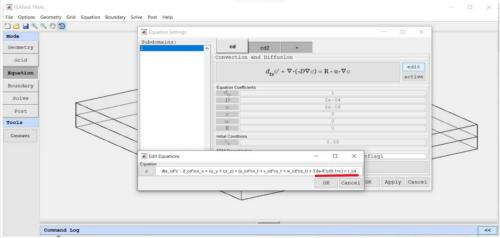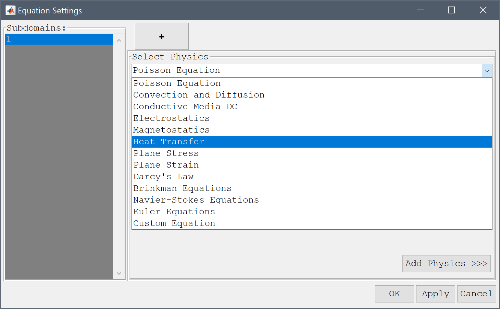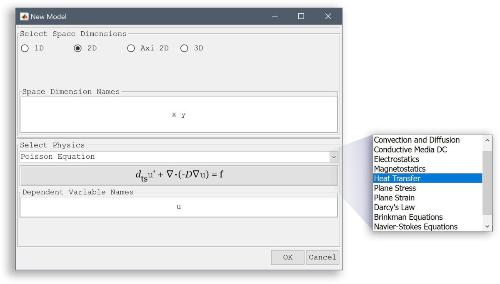Can one use multiple equations/dependent variables in FEATool?ClassicListThreaded1 messageJiao Zhao wrote Only one component can be set up in the equation (e.g. c shown in the equation Figure). Can we introduce multi-components in the equation?Multiphysics means you can add and couple more physics modes/equations using the + tab in the Equation Settings dialog box. https://www.featool.com/doc/physics.html#gui_mphysAlternatively, you can also create a *Custom Equation* PDE with any number of dependent variables by entering a space separated string (for example "c1 c2 c3") in the Dependent Variable Names edit field in the New Model dialog box: https://www.featool.com/doc/physics.html#phys_ce